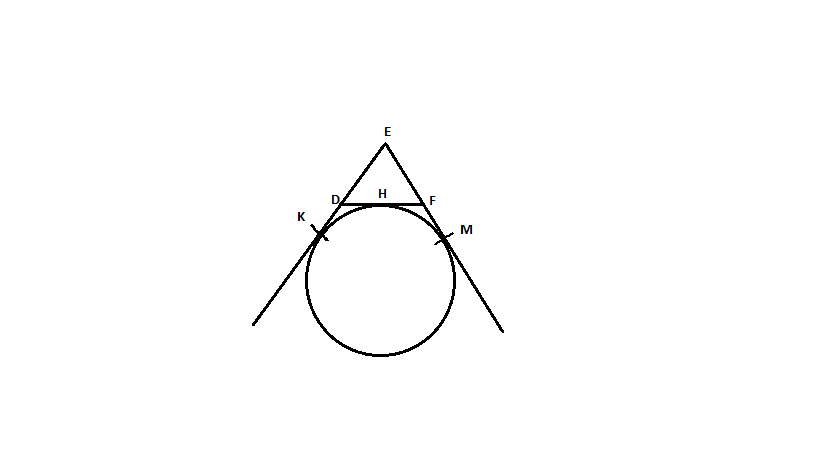QuestionAnswers

# In the figure, a circle touches the side DF of ∆EDF at H and touches ED and EF produced at K and M respectively. If EK = 9cm, then the perimeter of ∆EDF (in cm) is:A. 18B. 13.5C. 12D. 9Hint: EK and EM are tangents to the circle. Tangent segments to a circle from the same external point are congruent, (i.e. equal in lengths). Use this in finding the perimeter.

Given Data - A circle touches ED and EF at K and M respectively.
That makes EK and EM the tangents of the circle.
From the concepts of circles, we know that tangent segments to a circle from the same external point are congruent, (i.e. equal in lengths)
Given EK = 9cm
Therefore, EM = EK = 9cm (the exterior point is E)
Now, EK + EM = 18cm
We can write, EK = ED + DK and EM = EF + FM
⟹ED + DK + EF + FM = 18cm
Again from the exterior point D there are two tangents to the circle DK and DH and from exterior point F there are two tangents to the circle FH and FM.
Hence, DK = DH and FH = FM
⟹ED + DH + FH + EF = 18cm (FH = HF) -- These are interchangeable
We can write DH + HF = DF
⟹ED + DF + EF = 18 cm

The perimeter of a triangle = sum of all sides
The perimeter of a ∆ EDF = ED + DF + EF
= 18 cm

Note: In such problems having a complete understanding of the concept of circles is very important. Here we used the concept of tangents, where tangent segments to a circle from the same external point are congruent (i.e. equal in length).
A tangent from a given point to a circle is a straight line which touches the circle at only one point.
View Notes
Dark Side of the MoonSide of a PolygonConstruct Triangle Inscribed in a Circle